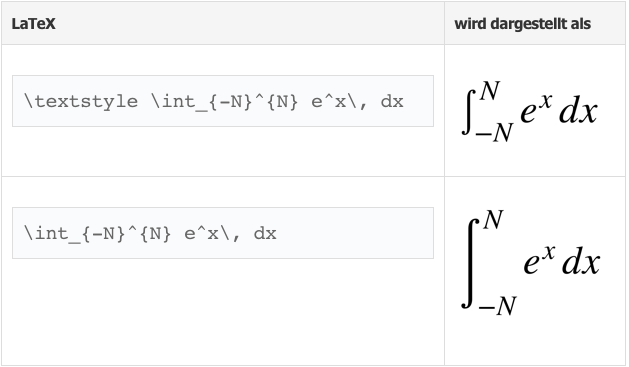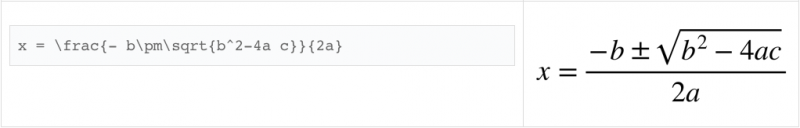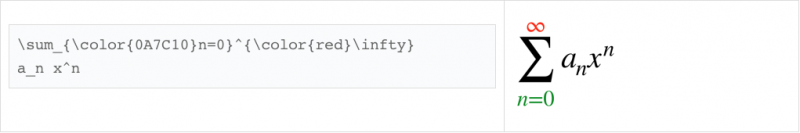# LaTeX What is shared

## Entering mathematics as a LaTeX script in class

With LaTeX, blind and sighted pupils can write math, chemistry and physics from the 5th grade up to the degree.

LaTeX is a line-based input from simple expressions to complex formulas that can be learned quickly.

A future-proof form of notation used worldwide - and not an isolated solution for the blind.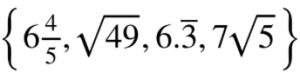### Crash course: Ready for lessons in 30 minutes (here as an example on the Apple Mac in Pages)

• Together with the studentOpen Pages and, if necessary, create a new document [cmd + N]. The whole thing can be reproduced in a similar way in Word on Mac and, somewhat more complicatedly, with Word on Windows.

(You should put as many commands as possible on the keyboard as shortcuts. You can do this in the VoiceOver utility under> Braille> Display)

1. ### Insert equation

• If the cursor flashes, you can use an equation instead of writing text, with [cmd + ⎇ + E]
• An input window appears.

Write

2 ^ 2 \ cdot5 = 20

say 2 to the power of 2 times 5 is 20

(^ stands for high and \ cdot stands for "center dot" or "Mal-Punkt". A backslash is made on the Mac with "alt + shift + 7")

In the same window you can see the result below - and have it read out with VO to check !! It will look like that: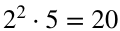Finished! That was pretty good! Now on "Insertclick and it's on the worksheet.

1. ### If the student wants to read, check, add to or change the equation with VO, he must go back to the input window!

A reading of the equation in the text with VO is NOT possible! Attention loss of time!

### This is how the student proceeds:

1. Place the cursor on an equation element (select using the cursor routing keys on the braille display), then
2. Press the trackpad with two fingers at the same time (corresponds to the right mouse button) to open the context menu (there is also a VO command for it that he / she may know).
3. Here he / she searches for the first entry with the arrow keys: "Edit equation" and comes into the input window.
4. After editing on "To update“Click and it is in the worksheet.

1. ### Special case

If you want to write several equations (only if there is an = in each one) in an input window. For example: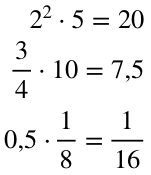Then the code looks like this:

\ begin {aligned} 2 ^ 2 \ cdot5 = 20 \ \ frac34 \ cdot 10 = 7.5 \ 0.5 \ cdot \ frac {1} {8} = \ frac {1} {16} \ end {aligned}

You only need the first and last lines if you want to write several equations in one window:

\ begin {aligned} x \ end {aligned}

The double backslash \ stands for new line in multiline equations

### Preparation of a math worksheet by the teacher

In order for the student to find the right task again, each task must have a heading. The headings should be created by the teacher. The student can then insert and find his equation under the heading!

Exercise 2:

Exercise 3:

### Learn the most important types of arithmetic here

If you need symbols that are not available on the keyboard or formulas that are not so easy to write, you can use special commands. Thesis start with a backslash (i.e. \). For example, \ le is the command which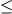generated.

\ frac xy (fraction) Fraction x through yx \ cdot y (center dot) x times yx \ ne y (not equal) x not equal to y \ sqrt  4 (square root) Square root of 4 \ sqrt [n ] {16} (square root) nth root of 16 \ sqrt {x + 2} (square root) square root of x + 2 \ pi (pi) Pi 4 ^ 2 (high) 4 to the power of 2 4 ^ { 12} (high) 4 to the power of 12 x \ le 8 (lower equal) x less than or equal to 8 a \ ge b (greater equal) a greater than or equal to b 30 ^ {\ circ} (circle) 30 degrees

The following table shows you some of the most commonly used commands in LaTeX

http://wiki.math.se/wikis/2009/bridgecourse1-TU-Berlin/index.php/5.1_Mathematische_Formeln_schreiben

### Mark with color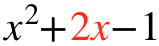{x ^ 2} + {\ color {red} 2x} -1

### Avoid common mistakes

One of the most common mistakes when editing math expressions is the $at the beginning and$ at the end.

Remember that commands start with a backslash (\) and add a space after the commands as long as they are not followed by a new command.

Another common mistake is to use an asterisk (*) instead of the correct symbol for multiplication (\ cdot in TeX).

### Superscripts and subscripts

For superscripts like potencies, one uses ^. For subscripts _. If the symbol to be superscripted or subscripted consists of more than one character, it must be in brackets {}.

### Fractions

As a rule of thumb, you should always write fractions as "small fractions" (i.e. with \ frac) if the numerator and denominator only consist of a few numbers and otherwise "large fractions" (i.e. with \ dfrac).

If an exponent or index contains a fraction, it should be written in one level for better readability, i.e.

5/2, and not as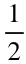### The most important basics in English:

http://www.malinc.se/math/latex/basiccodeen.php

### Examples to be amazed (for later)